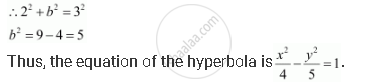CBSE (Arts) Class 11CBSE
Share
Notifications

View all notifications
Books Shortlist
Your shortlist is empty

# Find the Equation of the Hyperbola Satisfying the Give Conditions: Vertices (±2, 0), Foci (±3, 0) - CBSE (Arts) Class 11 - Mathematics

Login
Create free account

Forgot password?
ConceptHyperbola Eccentricity

#### Question

Find the equation of the hyperbola satisfying the give conditions: Vertices (±2, 0), foci (±3, 0)

#### Solution

Vertices (±2, 0), foci (±3, 0)

Here, the vertices are on the x-axis.

Therefore, the equation of the hyperbola is of the form x^2/a^2 - y^2/b^2 = 1.

Since the vertices are (±2, 0), = 2.

Since the foci are (±3, 0), c = 3.

We know that a2 + b2 = c2.Is there an error in this question or solution?

#### APPEARS IN

Solution Find the Equation of the Hyperbola Satisfying the Give Conditions: Vertices (±2, 0), Foci (±3, 0) Concept: Hyperbola - Eccentricity.
S